Shallow Foundation & Bearing Capacity

# Shallow Foundation & Bearing Capacity - Notes | Study Civil Engineering SSC JE (Technical) - Civil Engineering (CE)

 1 Crore+ students have signed up on EduRev. Have you?

Chapter 13
Shallow Foundation and Bearing Capacity:
FOUNDATION
Foundation is the lowest part of the building or the civil structure that is in direct contact with the soil which transfers loads from the structure to the soil safely. Generally, the foundation can be classified into two, namely shallow foundation and deep foundation.
1. Shallow foundation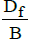<1
2. Deep Foundation>1.5

where, Df  is Depth of footing B is Width of footing
Types of Footing:
(A) Strip Footing or Continuous footing (L>>B):
-Provided for a row of columns which are closely spaced that their footings overlap each other.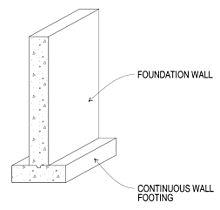(B) Combined Footing:
-Provided to support more than one column.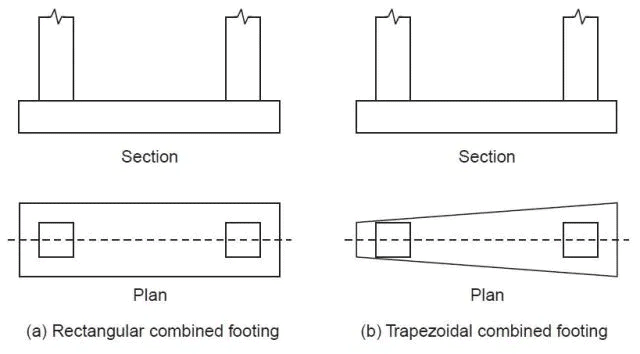(C) Spread Footing or isolated footing
-Provided to support an individual column.
-Circular,square and rectangular.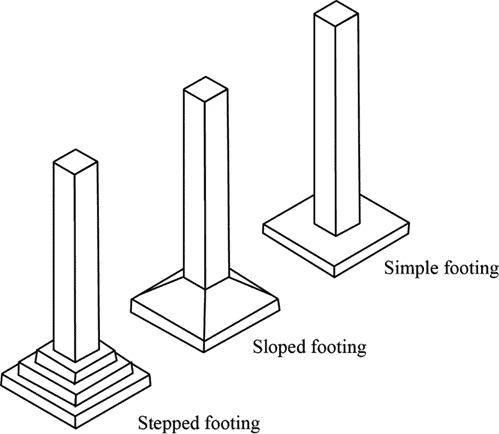(D) Raft Foundation:
-This is also called mat foundation.
-A  mat  foundation  consists  of  a  single  heavily  reinforced  concrete  slab  that  underlies  the  entire  structure  or  a  major  portion  of  the  structure.
-Mat  foundations  distribute  the  loads  over  a  large  area,  thus  reducing  the  intensity  of  contact  pressures.
-Structures   founded   on   relatively   weak   soils   may   be   supported   economically   on   mat   foundations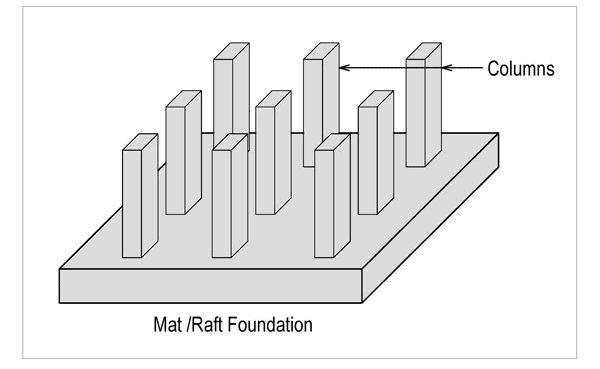BEARING CAPACITY:
It is the load carrying capacity of the soil.
Basic definitions:
-Ultimate bearing capacity or Gross bearing capacity (qu):  It  is  the  least gross  pressure which will cause shear failure of the supporting soil immediately below the footing.
-Net ultimate bearing capacity (qnu): It is the net pressure that can be applied to the footing by external loads  that  will  just  initiate  failure  in  the  underlying  soil.  It  is  equal  to  ultimate  bearing  capacity  minus  the stress  due  to  the  weight  of  the  footing  and  any  soil  or  surcharge  directly  above  it.  Assuming  the  density  ofthe footing (concrete) and soil (γ) are close enough to be considered equal, then
qnu = q- γDf
where,Df is the depth of the footing.
-Safe bearing capacity: It is the bearing capacity after applying the factor of safety (FS). These are of two types:
-Safe  net  bearing  capacity  (qns)  :  It  is  the  net  soil  pressure  which  can  be  safety  applied  to  the  soil considering only shear failure. It is given by,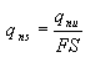-Safe gross bearing capacity (qs): It is the maximum gross pressure which the soil can carry safely without shear failure. It is given by,
q= qns + γDf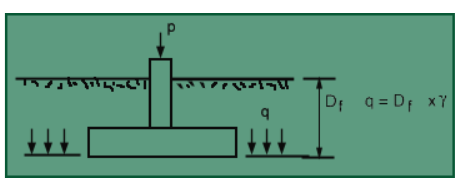Bearing capacity of footing

METHOD TO DETERMINE BEARING CAPACITY
(i) Rankines Method : Rankine (1885) attempted to determine ultimate bearing capacity of the soil by considering the equilibrium of two elements of the soil, one below the footing and another outside the footing adjacent to the first element.

Following is the equation for ultimate bearing capacity as per Rankine’s theory for cohesionless soil: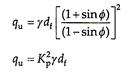where Kp is the Rankine’s coefficient of passive earth pressure.
As per Rankine’s theory, when the depth of foundation is zero, the ultimate bearing capacity is also zero, which is not true. As the Rankine’s theory does not give reliable value of ultimate bearing capacity, it is rarely used in practice. Instead, Rankine’s theory is used to determine the minimum depth of foundation as –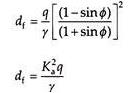where Ka is the Rankine’s coefficient of active earth pressure and q the maximum pressure applied at the base of the foundation.

(ii) Terzaghi's Method:
Terzaghi’s bearing capacity theory is useful to determine the bearing capacity of soils under a strip footing. This theory is only applicable to shallow foundations. He considered some assumptions which are as follows :
-The base of the strip footing is rough.
-The depth of footing is less than or equal to its breadth i.e., shallow footing.
-He neglected the shear strength of soil above the base of footing and replaced it with    uniform surcharge.(γDf)
-The load acting on the footing is uniformly distributed and is acting in vertical direction.
-He assumed that the length of the footing is infinite.
-He considered Mohr-coulomb equation as a governing factor for the shear strength of soil.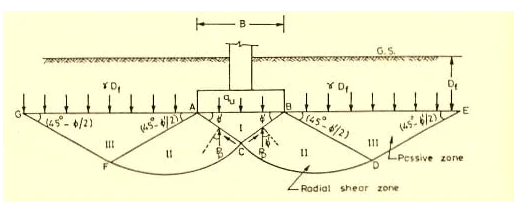For strip footing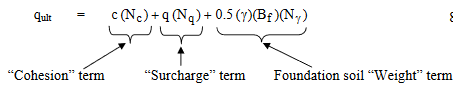where:
c = cohesion of the soil (kN/m2)
q = total surcharge at the base of the footing
= qappl + γa Df (kN/m2)
qappl = applied surcharge (kN/m2)
γa = unit  weight  of  the  overburden  material  above  the  base  of  the  footing causing the surcharge pressure (kN/m3)
D= depth of embedment (m)
γ = unit weight of the soil under the footing (kN/m3)
B= footing width, i.e., least lateral dimension of the footing (m)
N= bearing capacity factor for the “surcharge” term (dimensionless)
=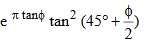N= bearing capacity factor for the “cohesion” term (dimensionless)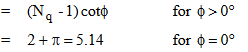Nγ = bearing capacity factor for the “weight” term (dimensionless)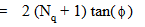Terzaghi has proposed certain shape factors to take care of the effect of the shape on the bearing capacity. The equation can be written as,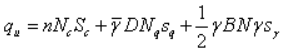where, sc,sq,sγ are the shape factors whose values for the square and circular footings are as follows
For long footings: sc,sq,sγ=1
For square footings: sc = 1.3, sq=1, sγ=0.8
For circular footings: sc = 1.3, sq=1, sγ=0.6
For rectangular footing of length L and width B: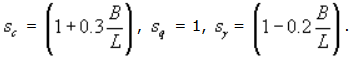(iii) Skemptons method (C-soil)
Skempton  (1951)  has  showed  that  the  bearing capacity  factors Nc in  Terzaghi's equation  tends  to  increase with depth for a cohesive soil.
For (Df/B)≤2.5,(where Df is the depth of footing and B is the base width)
(Nc) for rectangular footing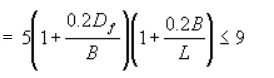For (Df/B)>2.5, Nc for rectangular footing =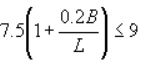(Nc) for circular and square footing =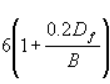The maximum value of Nc is 9.
This analysis is valid for any value of Df/B.
(iv) Meyerhof ’s Method:
- Bearing capacity for a srip footing at any depth.
qu = cNcscdcic + q0Nqsqdqi+ 0.5γBNγsγdγiγ
where s, d and i are shape, depth and inclination factor.
sc,sq,sγ = 1 for strip footing.
Nc,Nq,Nγ depends on the roughness of the footing, depth of the footing and the shape of the footing and the inclination of the loading in addition to the angle of shearing resistance phi.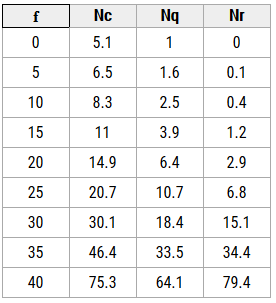Meyerhof’s bearing capacity factors

EFFECT OF WATER TABLE:
The position of ground water has a significant effect on the bearing capacity of soil. Presence of water table at a depth less than the width of the foundation from the foundation bottom will reduce the bearing capacity of the soil.
The bearing capacity equation incorporating the ground water table correction factors is given below.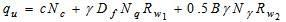Rw1 and Rw2 are water table correction factors
The water table correction factors can be obtained from the equations given below.
-When the water table is below the base of foundation at a distance ‘b’ the correction Rw2 is given by the following equation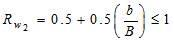when b=0,Rw2 = 0.5
-When water table further rises above base of foundation, correction factor Rw1  comes in to action, which is given by the following equation.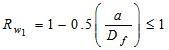when a=Df , Rw1=0.5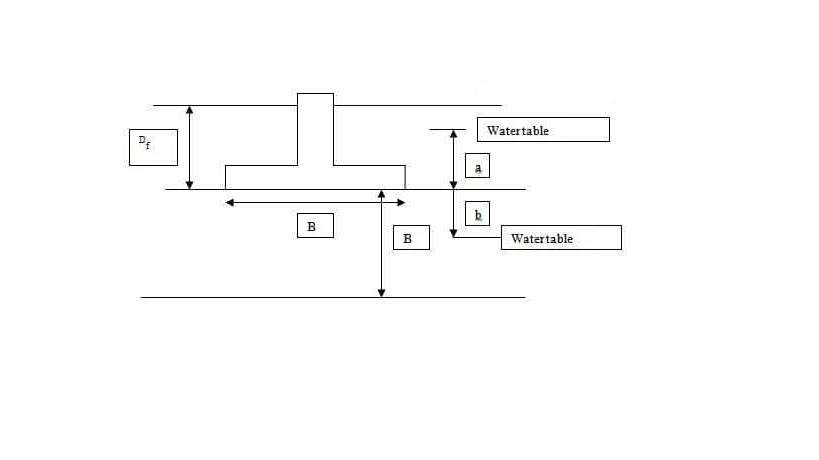Plate load test is done at site to determine the ultimate bearing capacity of soil and settlement of foundation under the loads for clayey and sandy soils. So, plate load test is helpful for the selection and design the foundation. To calculate safe bearing capacity suitable factor of safety is applied.
-Bearing Capacity Calculation for Clayey Soils
Ultimate bearing capacity = ultimate load for plate
quf = qup
-Bearing Capacity Calculation for Sandy Soils
quf/qup = Bf/Bp

Finally, safe bearing capacity = ultimate bearing capacity / factor of safety

The factor of safety ranges from 2 to 3.
-Foundation Settlement Calculation on Clayey Soils
Settlement of foundation (sf) = sp x Bf/Bp

-Foundation Settlement Calculation on Sandy Soils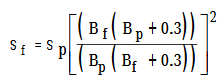where, quf = Ultimate bearing capacity of foundation
qup = Ultimate bearing capacity of plate
Sf = Settlement of foundations
Sp = Settlement of plate
Bf = Width of foundation
Bp =Width of plate

HOUSEL's APPROACH:
The method suggested by Housel for determining the safe bearing pressure on settlement
consideration is based on the following formula
Q=Apm+Ppn
where Q = load applied on a given plate, Ap = contact area of plate, Pp = perimeter of plate, m = a constant corresponding to the bearing pressure, n = another constant corresponding to perimeter shear.
The equation for a prototype foundation may be written as
Qf=mAf+nPf
where Af = area of the foundation, Pf = perimeter of the foundation.
When Af and Pf are known, the size of the foundation can be determined.

STANDARD PENETRATION TEST:
The standard penetration test is an in-situ test that is coming under the category of penetrometer tests. The standard penetration tests are carried out in borehole. The test will measure the resistance of the soil strata to the penetration undergone. A penetration emphirical correlation is derived between the soil properties and the penetration resistance.

Procedure for Standard Penetration Test:
-The test is conducted in a bore hole by means of a standard split spoon sampler.Once the drilling is done to the desired depth, the drilling tool is removed and the sampler is placed inside the bore hole.
-By means of a drop hammer of 63.5kg mass falling through a height of 750mm at the rate of 30 blows per minute, the sampler is driven into the soil. This is as per IS -2131:1963.
-The number of blows of hammer required to drive a depth  of 150mm is counted. Further it is driven by 150 mm and the blows are counted.
-Similarly, the sampler is once again further driven by 150mm and the number of blows recorded. The number of blows recorded for the first 150mm not taken into consideration.. The number of blows recorded for last two 150mm intervals are added to give the standard penetration number (N). In other words,
N = No. of blows required for 150mm penetration beyond seating drive of 150mm.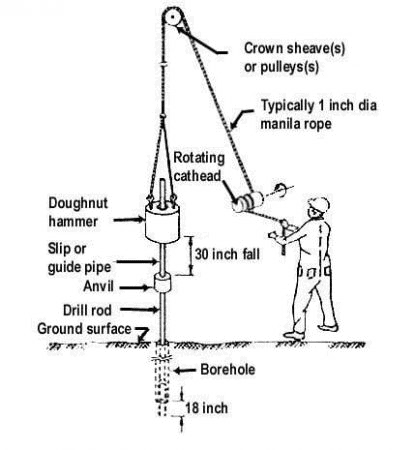If the number of blows for 150mm drive exceeds 50, it is taken as refusal and the test is discontinued. The standard penetration number is corrected for dilatancy correction and overburden correction.

Corrections in Standard Penetration Test:
Before the SPT values are used in empirical correlations and in design charts, the field ‘N’ value have to be corrected as per IS 2131 – 1981. The corrections are:
-Dilatancy Correction
-Overburden Pressure Correction
1. Dilatancy Correction
The corrected penetration number,
Nc = 15 + 0.5 (Nr -15)
Where Nr is the recorded value and Nc is the corrected value.
If Nr  less than or equal to 15, then Nc = Nr
2. Overburden Pressure Correction
The corrected value of ‘N’ is
Nc = CnN
Here Cn is the correction factor for the overburden pressure.

The document Shallow Foundation & Bearing Capacity - Notes | Study Civil Engineering SSC JE (Technical) - Civil Engineering (CE) is a part of the Civil Engineering (CE) Course Civil Engineering SSC JE (Technical).
All you need of Civil Engineering (CE) at this link: Civil Engineering (CE)

## Civil Engineering SSC JE (Technical)

2 videos|122 docs|50 tests
 Use Code STAYHOME200 and get INR 200 additional OFF

## Civil Engineering SSC JE (Technical)

2 videos|122 docs|50 tests

Track your progress, build streaks, highlight & save important lessons and more!

,

,

,

,

,

,

,

,

,

,

,

,

,

,

,

,

,

,

,

,

,

;